International Journal of Geomagnetism and Aeronomy
Vol. 4, No. 2, August 2003

# A special technique for testing models of continuous oscillatory processes in the magnetosphere

A. R. Polyakov and A. S. Potapov

Institute of Solar-Terrestrial Physics, Irkutsk, Russia

### Abstract

This paper offers a new method for processing and investigating regular oscillatory processes based on analyzing amplitude and phase fluctuations. The method makes it possible to determine (having the interval of a recording of the oscillatory process as initial information) the structure of differential equations controlling this process, as well as the numerical values of coefficients of all terms involved in them. The possibilities of the method are illustrated by considering geomagnetic Pc1 pulsations. It is shown experimentally that these pulsations, when observed on the ground, have properties which are consistent with that of forced oscillations of some resonating structure. The frequency of the main normal mode is estimated: f1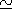0.2 Hz. Most likely the nature of this resonating structure relates to the ionosphere. It is also shown that within the framework of the adopted approach the second harmonic of the carrier frequency of Pc1 observed on the ground is caused by the second harmonic of magnetospheric radiation acting on the resonator and is not the consequence of the nonlinearity of the regime of forced oscillations.

### 1. Introduction

Wave and hydromagnetic diagnostics of the near-Earth plasma requires special methods to obtain information about the whole system, including parameters of the medium, basing on results of measurements made at some local site. There is no universal technique to achieve this goal. Different approaches are used depending upon the kind of oscillation, its source, and the structure of system. They include spectral analysis, the method of the analytical signal, study of dynamic spectra, examination of the dispersion, i.e. the frequency dependence of the arrival time of the signal, etc. By means of various tricks, these procedures provide individual characteristics of some parts of the system.

Here we present what is termed the technique of statistical modeling which is able to give information on general properties of an oscillating system, making it possible to build a mathematical model describing the dynamics of the system. Besides, this method provides a means of calculating, based on in-situ experimental measurements, some physical characteristics of the system, such as its eigenfrequencies and rate of decay. The technique suggested is based on analyzing the amplitude and phase fluctuations. Correlation functions of these fluctuations depend to a large measure on the properties of the subject under study thus providing the starting point for constructing a mathematical model of the object by solving an inverse problem. (However, we must note here that our technique does not follow the classic inverse theory quite strictly. Therefore, in this paper the term "inverse problem'' has conventional meaning.) This approach was first proposed by Gudzenko  and has been successfully used to study solar activity cycles [Gudzenko and Chertoprud, 1964]. In geophysics, it was used to investigate Pc4 [Guglielmi et al., 1983] and Pc3 [Polyakov and Potapov, 1989; Polyakov et al., 1992] continuous geomagnetic pulsations. It is known that Pc3 pulsations are forced oscillations of geomagnetic field shells. Therefore, to describe them, a model of a damped oscillator excited under the action of an external periodic force might be best suited for this purpose. Polyakov and Potapov  and Polyakov et al.  used the above model, and this allowed them to put forward a way to estimate such key parameters for diagnosing the magnetosphere as the damping coefficient and the oscillation eigenfrequency of various magnetic shells.

In this paper we give a description of a special technique for analyzing the oscillations (section 2), describe in details the procedure for solving an inverse problem (section 3), build a dynamic model of oscillations with taking into account the second harmonic of the carrying frequency (section 4), apply the statistical modeling technique to the analysis of Pc1 geomagnetic pulsation (section 5), and, in closing, discuss the results obtained (section 6).

### 2. Description of a Special Technique for Analyzing the Oscillations

Gudzenko  developed the algorithm for solving an inverse problem of statistical theory of oscillations. Being one of the ways to handle time series of continuous periodic processes, this algorithm makes it possible to reveal the properties and to determine quantitative characteristics of a model of the subject which gives rise to the oscillations. In the proposed method it plays the same role as Fourier-transform in spectral methods. Hence there is a need to consider its main concepts.

Any periodic oscillatory process with one degree of freedom x0(t) may be considered to be the solution of a set of ordinary differential equations of a general form: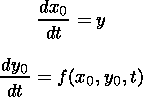(1)

Here f(x0,y0,t) is an arbitrary function only limited by the condition of x0(t) periodicity. The form of the dependence of this function upon variables determines the kind of oscillations: free, forced, etc.

Geomagnetic pulsations (ULF emissions), like most natural oscillatory processes, are not strictly periodic. Even within an ideal fragment of Pc type pulsations random changes of amplitude and phase inevitably occur. To take this into account, we add to the right-hand side of (1) a random function F(t). This can be done providing the amplitude and phase fluctuations are not large, i.e. F(t) does not heavily distort the output signal: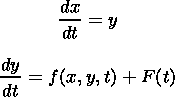(2)

Usually, a so-called Langeven source is used as a F(t), i.e. a random process, an auto-correlation function for which has the form of F(t)F(t+t)=2Dd(t), where d(t) is a delta-function, and D is the intensity of a random effect on the system. The technique proposed here does not require such a strong limitation. A sufficient condition is tFtF is the characteristic correlation time, and T is the oscillation period.

The model system of stochastic differential equations (2) forms the basis of the algorithm for solving an inverse problem. The procedures of this algorithm are all intended for determining the form of the dependence of a deterministic function f(x,y,t) upon its arguments. In doing so, a finite fragment of the measured signal x(t) is used as initial data, and scarcely any a priori information about the function f is invoked.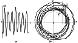Figure 1
Figure 1a gives an example of the solution of equation (2) for the case where f(x,y,t) represents forced oscillations: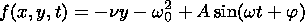(3)

where n and w0 are the damping coefficient and the eigenfrequency of the oscillator, respectively; A, w, and j are the amplitude, the frequency, and the initial phase of a periodic external force, respectively. The amplitude and phase of the solution contain fluctuations caused by the action of a random force F(t). In statistical radio physics, such a signal refers to periodic non-stationary random processes. For them, an extension of the ergodicity theorem [Gudzenko, 1961] is true. According to this theorem, an average (over the probability) oscillation is defined as an average of all separate oscillations which are present in the chosen time interval. We will designate an average over an ensemble of oscillations by the angle brackets. To define , let us now analyze the system of equations (2) on the phase plane by choosing x and y as Cartesian coordinates. With a small random action while D is less than the square of the signal amplitude an average solution (2) must coincide with a solution for the unperturbed system (1). Figure 1b presents a phase portrait of the solution (2), (3) corresponding to Figure 1a. Each separate oscillation x(t) determines a cyclic track in the phase plane, and an average solution x0(t), y0(t) corresponds to the mean statistical cycle shown in Figure 1b by the dotted line. Hereafter we will call this cycle the mean phase track (MPT). While the perturbation is small, the MPT represents a closed coil without self-crossings. A perpendicular to any point of the mean track intersects phase tracks at some points. The distances to these points are normal deviations of tracks from MPT. The position of the mean track in the phase plane is determined by the following condition: each of its points is the geometric sum of normal deviations. MPT is built by the oscillations phase portrait using a special method of successive approximations.

Let us now use a local system of coordinates related to MPT. We take at an arbitrary instant of time a point A on the phase track (see Figure 1b). For each of such points it is possible to determine uniquely the nearest point M at MPT. Let an interval AM be normal coordinate n in the local system, n=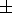AM, and the minus sign corresponds to points located inside MPT. Let the tangential coordinate q of the point A be defined as the length of the sector of MPT from the initial point M0 to the M projection measured in time units. For definiteness sake, it is assumed that the point M0 is the point where MPT intersects the negative ray of the horizontal axis X. Equations (1) for the mean phase track in local coordinates n and q take the formA random action F(t) in the system (2) leads to a deviation of phase tracks from MPT, therefore the following conditions must be true for them(4)

where g is a random value coinciding with the tangential deviation of the point A from MPT. Normal and tangential deviations have a simple physical meaning. They determine the amplitude and phase fluctuations, respectively, of the signal of unit frequency measured at the output of the system (2). The tangential coordinate q is the phase of the mean or non-disturbed oscillation, and t is the signal phase with taking into account the action by a random force F(t).

The local coordinates n and q completely describe the position of the point represented on the phase portrait of the oscillation providing the MPT is defined. Therefore in the set of equations (2) we can pass from the coordinates x and y to n and g , and oscillation phase t change to phase of MPT. To do this, we will use relations which follow from the sketch presented in Figure 1b:(5)

where a is the angle between a normal to MPT and the axis OY. On substituting equation (5) into equation (2) by taking into account equation (4), we obtain a set of equations which are linear with respect to the small values of n and g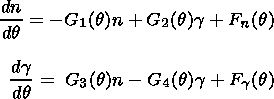(6)

Here Gi(q) are so-called dynamic coefficients (DC) which are defined by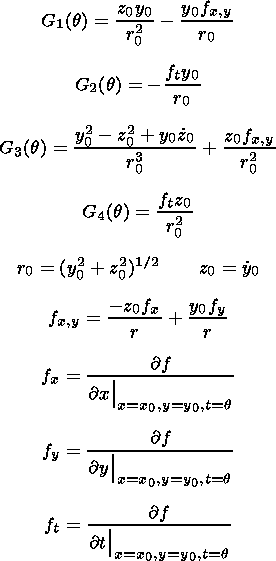(7)

The dot above a symbol means a derivative over q. Fn and F<FONT FACE='Symbol'>g are normal and tangential components of a random action, respectively,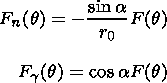(8)

It follows from equation (8) that DC are combinations of periodic functions. So they must also be periodic with the mean period of oscillations.

Simultaneous equations (6) represent a pair of nonhomogeneous linear differential first-order equations. Their solution can be written down in a general form using the concept of the transfer function H(q,t):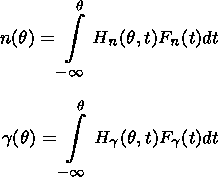(9)

Let us consider the first equation of the system (6). Let us multiply it successively by n(q -t ) and g (q - t ). After performing the procedure of averaging over an ensemble of phase cycles, we will have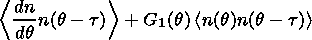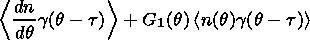(10)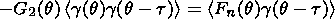Using the fact that the correlation time tF of the random function F is limited by the period, it is not difficult to show that the right-hand sides of the above equalities are zero providing t >tF. To do this, the relation (9) for n is substituted into the right-hand side of the first of the equalities (10). Taking into account (8), we obtain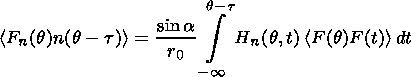We consider a random process F(t) to be a stationary process with a limited correlation time. This means that the correlation function under the integral depends only on the difference q -t and can be presented as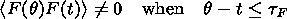Since t changes with integration from -to q -t the limits of the domain of variation of the function argument < F(q )F(t)> will beand t. If t >tF, then the integrand is zero with arbitrary t. We can prove in the same manner that < Fn(q )g (q -t )>=0 with t >tF.

If one applies the same procedure to the second equation of (6), then a set of four algebraic equations will result in which the dynamic coefficients Gi, i=1,2,3,4 are considered to be the unknown terms. By solving this set of equations, one gets formulas to determine the DC phase dependencies: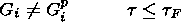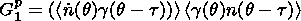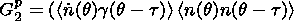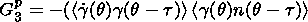(11)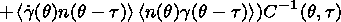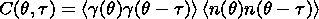It is necessary to note that Gip can be uniquely determined using the known fluctuations of the amplitude, phase, and their derivatives over the phase for an arbitrary value of t . If we find tF in any way, then we can determine the phase dependencies of DC which contains information about the form of dependence of the function f(x,y,t) on its arguments.

### 3. Procedure for Solving an Inverse Problem

The relations (11), along with the method of building MPT, are the basis for solving an inverse problem. The procedure of solving can be divided into several steps.

#### 3.1. Preprocessing

A fragment of the measured signal is chosen in such a way that the first and the last values both correspond to oscillation minima. Each of the values of the initial signal x(t) is normalized to the amplitude averaged over the fragment, and time t is measured in units of the mean period corresponding to the carrier frequency. After that, for every value of x(t), the value of y(t)=dx/dt is calculated.

#### 3.2. Constructing the Mean Phase Track

The tracks of all oscillations are plotted in the coordinate plane x,y. After that, the mean track is plotted by the method of successive approximations, and the local coordinate values of n and q are assigned to each of track points. The units of phase q measurement are radians, and n is a dimensionless quantity. Using formula (4) the tangential deviations are calculated. It is worth noting that MPT represents a parametric sequence of points (x0,y0). Thus it is easy to find the analytical form of the phase dependence of x0 as soon as the main track has been found in a graphic way.

#### 3.3. Searching for the Phase Dependence of Dynamic Coefficients

Correlation functions are calculated from the relations (11) for the main values of the phase q using the known values of n and g . The angle brackets designate an average over an ensemble of oscillations. So every correlator is defined as an average of those values of the quantity between the brackets that have a phase which differs from the main value by 2kp, where k is an integer. Formulae (11) can be only used when t >tF. The upper boundary for tF is the mean period, the lower boundary can be determined only by invoking additional information on the origin of oscillations. In the case of lack of such information every value of DC should be calculated for several different t , whereupon the average should be found in order to reduce the errors of DC evaluation. It should be noted that calculations of both the mean oscillation and DC are the more accurate, the greater number of oscillations is contained in the initial fragment of the signal. If this number is not very large (10-15), then the phase dependencies x0(q ) and Gi(q ) can differ substantially from the true ones.

#### 3.4. Revealing the Harmonic Structure of the Phase Dependence Functions x0(q ) and Gi(q )

These functions are found only for the main values of the phase, so they should be considered periodic. The frequency of the main mode, in view of the normalization, is unity. Since any periodic function can be represented as the sum of Fourier series, the following relations are true for the phase dependencies of x0 and Gi: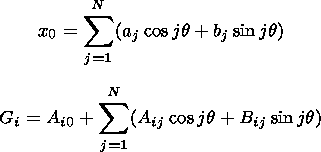(12)

Here j is the harmonic number equal to its frequency, i is the DC index, and N is the total number of harmonics. Amplitudes of the mean oscillation aj, bj are found when building MPT. The coefficients Aij, Bij can be calculated from the known dependencies Gi(q) by the method of least squares.

Thus the final product of the procedure of solving the inverse problem includes two groups of parameters, (aj,bj) and (Aij,Bij). The parameters of the first and second groups, respectively, characterize the mean oscillation and the deviation of actual oscillations from the mean. Consequently, no predetermined functional connection can exist between them, as it is impossible to indicate a universal procedure of calculating Aij,Bij from the known aj,bj, and vice versa. No such connection exists also between individual parameters inside each group. On the other hand, they must all depend, by definition, on the form of one function f(x,y,t). If it becomes clear after an independent calculation that some of them can be expressed in terms of others, then a study of these relationships must reveal the structure of the dependence of the function f on its argument.

Proceeding further along the path of solving the inverse problem, we seek to find the method of determining f(x,y,t) from the known aj,bj,Aij,Bij. To do this, we consider the case of forced oscillations as an example. The system (2), (3) should be considered to be the initial equations describing these oscillations. Upon substituting (3) into (7), we determine the form of the phase dependencies of the DCs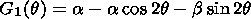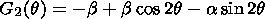(13)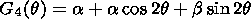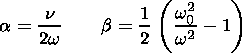By comparing (12) and (13), it is easy to check that the output parameters of the inverse problem for forced oscillations are related by the following relations: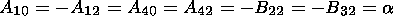(14)The MPT, in view of the normalization, will have the form of a circle of unit radius. Hence the output parameters of the mean oscillation may be represented as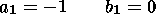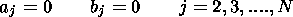Thus, if the output parameters of the inverse problem for the oscillatory process satisfy the conditions (14), then they will be forced oscillations, and the dynamics of the process must be described by the system of equations (2), (3). Using such an approach, it was shown in [Polyakov and Potapov, 1989] that geomagnetic pulsations Pc3 refer to this type of oscillations. This conclusion could be considered trivial, if, along with it, there were no possibility of experimentally determining such parameters of the oscillator as the damping coefficient n and the eigenfrequency w0. Formulas by which these parameters are calculated, are the consequence of the conditions (14) and have the form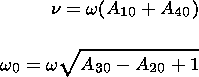(15)

Consider now the case of weakly nonlinear forced oscillations. Assume that the right-hand side of (3) additionally contains small (in value) nonlinear second-order terms c1x2,c2y2,c3xy. The MPT in this case does not have the form of an ideal circle, because the phase dependence of the mean oscillation contains a small (in amplitude) second harmonic a2 0, b2 0. By making use of the relations (7), it is possible to demonstrate that in the phase dependencies G1(q ) and G3(q ) (13) there appear terms corresponding to the fundamental and third harmonics. As a result, the conditions (14) are supplemented by the relations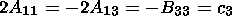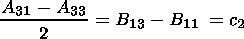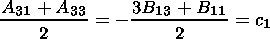It should be noticed that c1,c2,c3 are dimensionless quantities, because the arguments of the function f(x,y,t) are considered normalized to the mean amplitude and to the mean period. It has been pointed out above that the accuracy of determining the output parameters of the inverse problem depends on the number of oscillation periods containing in the sample. Therefore, we verified the resulting conditions not through a simple comparison of the output parameters but using statistical methods of verifying hypotheses. Fulfillment of the conditions serves as the proof that the relation for the function f(x,y,t) involves nonlinear terms. By calculating the coefficients c1,c2,c3 from the output parameters, we thereby determine the form of each of them.

Harmonics in forced oscillations can appear not only due to nonlinear terms in equations (2), (3). A similar result ensues from the presence of small oscillations in the driving force which have a frequency multiple to the carrier frequency. However, if we are dealing with the second harmonic of the driving force, then the additional fundamental and third harmonics in the phase dependencies (13) appear in another pair of coefficients G2(q ), G4(q ). By analyzing the relationships between output parameters of these harmonics, we can either accept or discard a hypothesis of presence of oscillations with double frequencies in the source, as well as determine the numerical values of the characteristics of these oscillations. In this way, using the proposed method of analysis, it is possible to exactly indicate the cause of the appearance of harmonics in the oscillations under investigation.

The oscillations that contain the third harmonic are investigated according to the same scheme. The difference from the preceding case only implies that the phase dependencies of the DCs (13) are supplemented not by the fundamental and third harmonics, but by the second and fourth harmonics. Such a result suggests an important generalizing conclusion. The terms of a different power index in the arguments x and y involved in the expression for the function f(x,y,t) contribute to different harmonics of phase dependencies of dynamic coefficients. For that reason, by analyzing the harmonic composition of the DCs, we can determine the form of all terms in the function f and calculate the values of dimensionless coefficients involved in each of them. This permits us to achieve the final goal of the proposed method: to construct, from observed oscillations, a system of equations, the solution of which is provided by these oscillations themselves.

### 4. General Dynamic Model of Oscillations With Provision for the Second Harmonic of the Carrier Frequency

We now consider in greater detail the situation where the presence of the second harmonic in the oscillations under investigation is caused simultaneously by the two factors indicated above. In this case, for the function f(x,y,t), in view of the normalization at the first stage of the solution of the inverse problem, we have, instead of (3),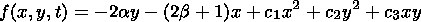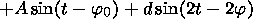(16)The nonlinear contribution to this function is determined by three dimensionless coefficients c1,c2,c3, and the second harmonic of the source is characterized by the amplitude d and by the initial phase 2j . The mean solution x0 of the system of equations (2), (16) can be found by the method of successive approximations by assuming that the above parameters are small in magnitude. Relations for the coefficients of the Fourier series (12) to a first approximation have the form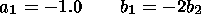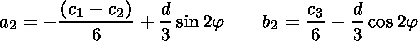Upon substituting x0(q ) into (7), in view of (16), we obtain formulas for the phase dependencies of the DC Gi(q ). Since these dependencies are defined by harmonic functions, it is an easy matter to obtain from them the relations for the output parameters of the inverse problem Aij,Bij. To a linear (in the small parameters c1,c2,c3,d ) approximation, they are conveniently represented as the Table 1. Examination of Table 1 shows that the output parameters with the harmonic numbers j = 0,2 are related by the same relations as in (14). This means that these harmonics of the DCs are defined solely by the linear part of the function (16) and by the main mode of the source. At the same time, nonlinear terms and the second harmonic of the source make a contribution to the fundamental and third harmonics of the DCs. Furthermore, in the DCs with the numbers i = 2, 4, as is evident from the table, the fundamental and third harmonics are caused by the presence or absence of the double frequency in the source spectrum.

The resulting relations can be taken as the basis for continuing the solution of the inverse problem. Indeed, the final product of the procedure of processing the initial signal, as shown above, is represented by the parameters Aij and Bij. Using them, as well as the formulas from Table 1, it is easy to determine the coefficients of the function (16). In such a case, the output parameters of the inverse problem can be considered to be the parameters a,b,c1,c2,c3,d,j characterizing the form of a function which mathematically describes, together with the system (2), the deterministic behavior of the object that generates oscillations. It is worthwhile to note an important (in our view) property of this stage of the inverse problem. It follows from the formulas of Table 1 that each desired parameter can be expressed simultaneously in terms of different Aij,Bij which are calculated in the procedures of the inverse problem independently from each other. This makes it possible not only to calculate the values of the parameters but also to establish the fact of presence or absence of some or other term in the function (16). Let us consider an example of the output parameters a and b . To each of them we put in correspondence two parameters such that one of them is determined from dynamic coefficients with the numbers i = 1,3, and the other from the DCs with i = 2,4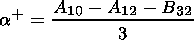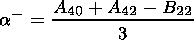(17)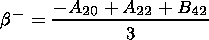Through a simple substitution it is easy to check that if Aij,Bij are defined by the formulas from Table 1 which depend only on the form of the function f(x,y,t), then the parameters in the chosen pairs coincide. This suggests an important (for the inverse problem) conclusion: the coincidence of the parameters in the pairs is a necessary indication that the linear dependence on the arguments of f(x,y,t) is the same as in (16) and that the inhomogeneity of the function is caused by the harmonic source. To put it a different way, the oscillations under investigation should be referred to the type of forced oscillations of the attenuating oscillator. In order for this indication to be, in addition to necessary, also sufficient, one should prove that for the other types of oscillations the parameters in the pair of (17) must not coincide. Consider free oscillations and self-oscillations. They are characterized by the homogeneity of the unperturbed system of equations (1). This means that the function f does not depend on time explicitly, and its partial derivative ft is zero. Taking into account this factor in the relations (7), it is easy to check that the dynamic coefficients G2 and G4 identically equal to zero. Since the second parameters in (17) are determined from these DCs, it is obvious that a-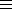b-0. At the same time the first parameters depend on the zeroth and second harmonics G1,G3 which are caused by linear terms in the function f(x,y). They must have the same form as in the case of forced oscillations if dissipative processes are taken into account, which are inherent in any natural system. Hence the first parameters in the pairs of (17) are determined by the formulas from the table and depend on a and b . The frequency mismatch b for the types of oscillations under consideration is nonzero, because in free oscillations the carrier frequency differs from the eigenfrequency by the contribution from the damping factor and in self-oscillations by nonlinear corrections. Consequently, a+ 0, b+ 0, which indicates the noncoincidence of the coefficients in the pairs of (17) for free oscillations and self-oscillations. As far as parametric oscillations are concerned, the function f for them is explicitly time-dependent. However, it follows from the relations (7) that this dependence does not make any contribution to the zeroth and second harmonics of the dynamic coefficients G2,G4. Hence, as in the preceding case, the parameters in the pairs must not coincide. Thus the considerations presented above permit us to formulate the final conclusion: the coincidence of the parameters of the inverse problem in the pairs of (17) is a necessary and sufficient indication of the fact that the oscillations under investigation refer to the type of forced oscillations of an attenuating oscillator.

For each of the remaining output parameters c1, c2, c3, d cosj, d sinj, we arrange in a similar manner each own pair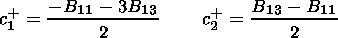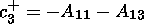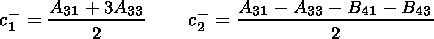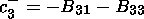(18)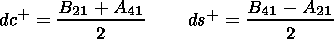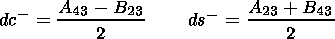For them, as done for (17), we can prove that the coincidence of the parameters in any one of the pairs can serve as a reliable indication of the fact that the function (16) involves a term proportional to the corresponding output parameter. This means that if, for example, the parameters c1+, c1- obtained by processing the oscillatory process using the procedures of the inverse problem are found to be identical, then the term c1x2 must be involved in the function f(x,y,t) of model equations (2) for these oscillations.

Thus our proposed method provides a means to reconstruct the function f(x,y,t) from the output signal. However, its practical implementation involves certain difficulty which should be taken into consideration. The point here is that, as shown above, the output parameters Aij and Bij are calculated in inverse problem procedures with a certain uncertainty which increases with a decrease of the number of oscillations in the output signal interval that is processed. For regular geomagnetic pulsations, even in the most favorable stationary regime of generation, it is difficult to detect the interval containing more than a few tens of oscillations. The parameters in the pairs of (17), (18) in this case become, to a certain extent, random quantities, and their simple algebraic comparison loses its meaning. Rather, it is necessary to use comparison rules employed for random numbers [see, e.g., Hudson, 1964]. To do so, one should select and process at a time many intervals of oscillations referring to a single process. This will make it possible to create a sample of random quantities (17), (18) which can be used to determine the correlation coefficients K of the parameters of each pair. It follows from formulas (17), (18) that whenever the parameters in a pair must coincide, they are both equal to one of the output parameters. This means that a linear functional connection occurs between them. The correlation coefficient in such a case must assume values close to unity. And, on the contrary, if the numbers in a pair are defined by different formulas, then a change of one of them is in no way associated with a change of the other. Consequently, no functional connection exists between them, and the correlation coefficient must be zero. Thus large values of K can be chosen as one of the criteria of coincidence of the parameters in the pair. We now introduce a generalized designation a and b for the numbers in the pair. If input information for the solution of the inverse problem is provided by a limited, selective interval of the oscillatory process, then each of them can be represented as the sum of the determined and random terms a=d+ a, b=d+ b, where d is a general designation of the corresponding output parameters, and a and b are statistically independent random numbers. It is obvious that if d = 0, then for such a pair K = 0. If the situation is the reverse, i.e. when a= b=0, we have K = 1. This means that if the error of determining the parameters of the pair by far exceeds the value of the output parameter corresponding to this pair, then the correlation coefficient between them can be not very high even in the case where determined terms in each of them are identical. Therefore, as one further criterion, we chose the quantity T characterizing the ratio of the parameters of the pair which is determined by the formula T = 2ab/(a2 + b2). It is easy to understand that in the case of a statistical coincidence of a and b the distribution of T must have a maximum when T = 1. Thus, if this condition is satisfied and, besides, if the correlation coefficient has a value close to unity, it can be stated with confidence that the parameters of the pair of random numbers are statistically identical.

To conclude section 4, we discuss some details of the proposed method of fluctuation modeling of oscillatory processes. The relations (17) give us indications which permit us to determine the cause of the generation and the dynamics of development of the oscillatory process, i.e. they determine the type of oscillations. Indeed, if the parameters in the pairs coincide, then the oscillations should be considered forced. If, however, no coincidence is observed, then using the scheme of reasoning described above, one can form similar pairs of parameters for other types of oscillations (parametrical, self-oscillations, etc.). Upon determining in which particular pairs the parameters are identical, we obtain the answer to the question: To what type do the observed oscillations refer? This, in turn, establishes automatically the basic, linear structure of the function f(x,y,t) of model equations (2). As soon as the form of the function has been established, it is easy to determine the numerical values of the coefficients of its terms. Note that these coefficients can not always be the final goal of the proposed method. Often, especially in problems of investigating the new properties of geomagnetic pulsations, the more important issue is to determine the type of oscillations and the structure of the desired function. As far as the relations (18) are concerned, they, unlike (17), give indications of the presence or absence of terms of the function which distort only slightly the form of the output signal. They can be involved in the function f(x,y,t) (and may not be involved) for oscillatory processes of any type. All depends on whether the parameters of the corresponding pair of (18) coincide or not.

### 5. Application of a Statistical Modeling Technique to the Analysis of Pc1 Geomagnetic Pulsation

To test the effectiveness of the method described above, we chose geomagnetic Pc1 pulsations as the object of study, because records just of these pulsations can provide favorable material for a processing. The properties and characteristics of Pc1 are given, for example, by Guglielmi and Pokhotelov . Mention should be made also of Alpert and Fligel , who found small spectral multiples to the carrying frequency in Pc1 spectrum. A phase-portrait method was used to investigate a second harmonic of Pc1 spectrum by Kiselev and Kozlovskii .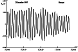Figure 2
When selecting the intervals suitable for a processing, we used records of the geomagnetic field in the frequency range 0.2-3.0 Hz from station Batagai, East Siberia, Russia ( F = 57o, L =191o ). The selection criteria are rather simple and follow from the main principle of the method used: pulsations in the interval selected for the analysis must not contain amplitude and phase slips, and must include as many oscillations as possible, because this determines the accuracy of determining the output parameters of an inverse problem. Oscillation slip means a small-scale kink at the phase plane. For Pc1 pulsations their amplitude modulation is the characteristic feature. However, providing amplitude changes are slow as compared to the oscillation period this modulation has little or no effect on the output parameters. An example of such a suitable interval is illustrated by Figure 2.

By adhering to this criterion, we selected about 150 intervals of Pc1 pulsations, each of which contained from 10 to 20 oscillations. A preprocessing implied digitizing magnetic analog records with the sampling rate of 20 Hz. Subsequently, the numerical set of each interval was processed sequentially, following all procedures of the method that has been described in detail in the first part of this paper. As the output result for each interval of Pc1, we obtained 28 values of the parameters Aij, i=1,2,3,4 j=0,1,2,3; Bij, i=1,2,3,4 j=1,2,3. From them, using the relations (18) and (19) we formed samples of pairs of numbers corresponding to the output parameters a,b,c1,c2,c3,d cosj,d sinj. Each sample has the size equal to the number of processed intervals. Finally, for each pair we determined the correlation coefficient K between its parameters, and the array of values of the quantity T which, it must be recalled, characterizes the ratio of the parameters of the pair. In what follows, in order to distinguish these characteristics for different pairs, we shall assign to them the lower index of the corresponding output parameter. These characteristics must permit us to determine in which pairs the parameters are statistically identical and in which they are not identical, and on this basis, to draw the conclusion about the structure of the function f of governing model equations (2) for Pc1 pulsations.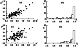Figure 3
We shall analyze the results obtained starting from the parameters defined by (17). For illustrative purposes, Figures 3a and 3c plot the mutual dependencies of the parameters in the pairs. One can notice that the position of points on both plots indicates a rather high degree of linear dependence of one parameters on the other. This is confirmed by the values of the correlation coefficients K<FONT FACE='Symbol'>b = 0.87 and K<FONT FACE='Symbol'>a = 0.66. Besides, the distributions of T<FONT FACE='Symbol'>b and T<FONT FACE='Symbol'>a , plotted in Figures 3b and 3d, have clear maxima corresponding to the values of T<FONT FACE='Symbol'>a=1.0 and T<FONT FACE='Symbol'>b=1.0. All this indicates that the parameters in the pairs of (17) statistically coincide for the sample of Pc1 pulsations under consideration. Consequently, the necessary and sufficient condition is thereby satisfied, according to which these pulsations should be referred to the type of forced oscillations of an attenuating oscillator.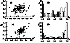Figure 4
Let us consider the results of calculations for the pairs of (18) which are responsible for the presence or absence of nonlinear terms in the function f. Since the parameters of these pairs are found to have similar properties, we use only one of them in the analysis. Figure 4a plots c1+ versus c1-. This dependence represents an ensemble of uniformly arranged points which does not have any well-defined direction. The correlation coefficient for this pair K c1 = 0.13. For the other pairs, it has also small (in magnitude) values Kc2=- 0.11 and Kc3= 0.25. The distribution of Tc1, plotted in Figure 4b, is almost uniform and does not have a clear maximum at all. This means that the parameters in the pairs under consideration do not coincide statistically and are independent random quantities caused by the error in calculating the output parameters of the inverse problem. Consequently, nonlinear terms in the function f are absent in the system of governing equations (2) for Pc1 pulsations.

The conclusion drawn here means that the second harmonic of the carrier frequency involved in Pc1 pulsations, if it exists, must be caused by the second harmonic in the source. To check that this is the case, we now consider the results of calculations for the two last pairs in (18). The distribution of Tdc, as is evident from Figure 4d, has a sufficiently clear maximum around unity, and the dependence of dc+ on dc-, plotted in Figure 4c, is characterized by the correlation coefficient Kdc= 0.63. For the pair corresponding to the output parameter d sinf , the results obtained were similar, because the peak of the distribution of Tdc lies near unity, Kds= 0.58. Note that not so high (as one would like) values of the correlation coefficients can be caused, as shown above, by the small value of the parameter d, even when the parameters in the pairs coincide statistically. Refined criteria of coincidence in this case are represented by peaks in the distributions of Tdc and Tds. Since these distributions have clear maxima and, besides, the values of Kdc and Kds are indeed much higher than the correlation coefficients of the first three pairs of (18), we can be confident that the parameters in the pairs under consideration are statistically identical. This means that the source of Pc1 pulsations has a small (in amplitude) second harmonic. The result obtained contradicts to the one presented by Kiselev and Kozlovskii , who related the Pc1 second harmonic to wave propagation in a non-linear medium.

### 6. Discussion of Results

So, in sections 2-5 it was shown that Pc1 pulsations can be well described by the model of attenuating oscillator which is presented by the system of equations (2) and (16) with c1=c2=c3=0. At this point, however, it is necessary to point out an important factor. Strictly speaking, the model of oscillator describes the systems with lumped parameters like a pendulum or an oscillating circuit. In the near-terrestrial plasma we are concerned mainly with systems having distributed parameters. For such systems a resonator or waveguide are the analogues of oscillator. Inasmuch as Pc1 pulsations are generated and propagated in the magnetosphere and ionosphere they would be more properly classified as forced oscillations of a resonator or a waveguide. At the present time we are developing a model of resonator with attenuation on which to analyze in detail fluctuation characteristics of Pc1. First, positive results have been obtained already. However we are confident that preliminary conclusions on the properties of a waveguide or resonator which forms Pc1 features can be made just now starting from the oscillator model (2),(16) and considering the eigenfrequency involved in the coefficient b as just one value from the infinite series of the oscillatory normal mode frequencies.Figure 5
Let us try to find out what frequencies of normal modes are specific to a resonant structure that imprints on fluctuation properties of Pc1 oscillations. To do this, using the relations (15) we calculated the values of w0 for all intervals of the sample. Results are presented in Figure 5b in the form of a histogram of the distribution. For comparison, Figure 5a plots the distribution of observed frequencies w . Note that when selecting the intervals, their frequency was in no way specially checked upon. As may be seen from the figure, the frequency values broke up into two groups: high-frequency, and low-frequency. It can be noticed, however, that relative changes of the number of cases for different frequencies are small, i.e. the distribution in groups, especially in the low-frequency one, can be considered uniform. In the w0 distribution, however, three equally spaced peaks are evident. This can mean that the peaks correspond to normal modes of the required resonator or waveguide, and the interval between neighboring frequencies Df is about 0.2 Hz. Let us assume that the spectrum of normal mode frequencies is equidistant, fn = n f1. If so, a relation df = fn+1 - fn = f1 is bound to hold for all harmonic numbers n. Then we can make an estimate of the main mode frequency of the resonator or waveguide (its fundamental frequency): f1=Df0.2 Hz. Such a value is obviously too high for the first harmonic frequency of the magnetic shell which can be considered as the resonator for ion cyclotron waves [see Alpert and Fligel, 1985]. At the same time it is in good agreement with the cut-off frequency of ionospheric waveguide. It is unlikely that the obtained fundamental frequency can be connected with the classical waveguide formed by F2 layer of the ionosphere [Manchester, 1970] because it is a refractive waveguide, it can not hold waves propagating at normal angle to its axis. So it has a cut-off frequency, but has not a fundamental one. However, the literature contains examples of other resonant structures in the ionosphere like the ionospheric Alfvén resonator [Demekhov et al., 2000, Pokhotelov et al., 2001], which can execute forced oscillations recorded as Pc1.

### 7. Conclusion

The main conclusions of this study may be summarized as follows:

1. We have suggested a new method for investigating stationary oscillatory processes based on analyzing amplitude and phase fluctuations. The method makes it possible to determine (having the interval of a recording of the oscillatory process as initial information) the structure of differential equations controlling this process, as well as the numerical values of coefficients of all terms involved in them.

2. The possibilities of the method are illustrated by considering geomagnetic Pc1 pulsations. It is shown experimentally that these pulsations, when observed on the ground, have properties which are consistent with that of forced oscillations of some resonating structure. The frequency of the main normal mode is determined: f10.2 Hz. Most likely the nature of this resonating structure relates to the ionosphere.

3. It has been found that within the framework of the adopted approach the second harmonic of the carrier frequency of Pc1 observed on the ground is caused by the second harmonic of magnetospheric emission acting on the waveguide and is not the consequence of the nonlinear regime of forced oscillations. Master equations for these pulsations should be considered to be the system (2), (16), in which it is necessary to take into consideration that c1=c2=c3=0.

#### Acknowledgments

We are grateful to A. V. Guglielmi for interest to the work and useful comments. This work was done with financial support from the Russian Foundation for Basic Research (grant 00-05-64545) and INTAS Foundation (grant INTAS-01-0013).

### References

Alpert, Ia. L., and D. S. Fligel, On Fourier-analysis of geomagnetic pulsations Pc1, "two-fold" and "three-fold" pulsations Pc1 and fundamental frequencies of the magnetospheric cavity. I,  Planet. Space Sci., 33, 993, 1985.

Demekhov, A. G., V. Yu. Trakhtengerts, and T. Bösinger, Pc1 waves and ionospheric Alfvén resonator: Generation or filtration? Geophys. Res. Lett., 27, 3805, 2000.

Gudzenko, L. I., The generalization of an ergodic system to nonstationary random processes, Radiophysics (in Russian), 4, 267, 1961.

Gudzenko, L. I., A statistical method for determining the characteristics of a noncontrolled self-oscillatory system, Radiophysics (in Russian), 5, 572, 1962.

Gudzenko, L. I., and V. E. Chertoprud, Some dynamic properties of cyclic activity of the Sun, Astron. Zh. XLI(4), 697, 1964.

Guglielmi, A. V. and O. A. Pokhotelov, Geoelectromagnetic Waves, Inst. of Phys., Philadelphia, Pa., 1996.

Guglielmi, A. V., B. I. Klain, and A. R. Polyakov, Dynamic parameters of the self-oscillation model of geomagnetic pulsations, Geomagn. Aeron. (in Russian), 23, 630, 1983.

Hudson, D., Statistics. Lectures on Elementary Statistics and Probability, Geneva, 1964.

Kiselev, B. V., and A. E. Kozlovskii, Quasi-harmonic character of Pc1 geomagnetic pulsations, Geomagn. Aeron. (in Russian), 29, 748, 1989.

Manchester, R. N., Propagation of hydromagnetic emissions in the ionospheric duct, Planet. Space Sci., 18, 299, 1970.

Pokhotelov, O. A., V. Khruschev, M. Parrot, S. Senchenkov, and V. P. Pavlenko, Ionospheric Alfvén resonator revisited: Feedback instability, J. Geophys. Res., 106, 25,813, 2001.

Polyakov, A. R., and A. S. Potapov, Dynamic parameters of geomagnetic Pc3 pulsations, Geomagn. Aeron. (in Russian), 29, 921, 1989.

Polyakov, A. R., A. S. Potapov, and B. Tsegmed, Experimental estimation of the resonance frequency and quality of mid-latitude Alfvén resonators, Geomagn. Aeron. (in Russian), 32, 156, 1992.

Load files for printing and local use. This document was generated by TeXWeb (Win32, v.1.3) on August 8, 2003.